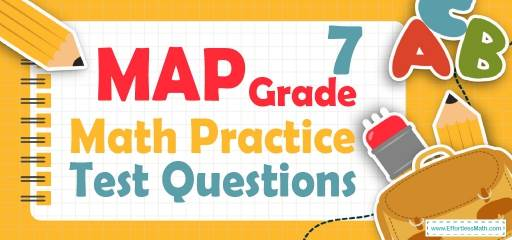# 7th Grade MAP Math Practice Test QuestionsSuccess in the 7th Grade MAP Math test is possible with high speed of operation and reduction of carelessness. Achieving this level of ability is possible with enough practice. 7th Grade Students need to know that they should not just study because studying without practice will not be useful at all. Therefore, here we provide a step-by-step guide to solving 10 of the 7th Grade MAP Math Practice Test questions that cover the most important mathematical concepts in the 7th Grade MAP Math test.

Make sure to follow some of the related links at the bottom of this post to get a better idea of what kind of mathematics questions students need to practice.

## 10 Sample 7th Grade MAP Math Practice Questions

1- A chemical solution contains $$4\%$$ alcohol. If there is 24 ml of alcohol, what is the volume of the solution?

A. 240 ml

B. 480 ml

C. 600 ml

D. 1200 ml

2- The price of a laptop is decreased by $$10\%$$ to $360. What is its original price? A. 320 B. 380 C. 400 D. 450 3- What is the median of these numbers? $$4, 9, 13, 8, 15, 18, 5$$ A. 8 B. 9 C. 13 D. 15 4- Three times the price of a laptop is equal to five times the price of a computer. If the price of the laptop is$200 more than the computer, what is the price of the computer?

A. 300

B. 500

C. 800

D. 1500

5- What is the perimeter of a square that has an area of 595.36 feet? ________

6- Jason is 9 miles ahead of Joe running at 5.5 miles per hour and Joe is running at the speed of 7 miles per hour. How long does it take Joe to catch Jason?

A. 3 hours

B. 4 hours

C. 6 hours

D. 8 hours

7- 55 students took an exam and 11 of them failed. What percent of the students passed the exam?

A. $$20 \%$$

B. $$40 \%$$

C. $$60 \%$$

D. $$80 \%$$

8- Jason needs an $$75\%$$ average in his writing class to pass. On his first 4 exams, he earned scores of $$68\%, 72\%, 85\%$$, and $$90\%$$. What is the minimum score Jason can earn on his fifth and final test to pass? __________

9- A bank is offering $$3.5\%$$ simple interest on a savings account. If you deposit $12,000, how much interest will you earn in two years? A.$420

B. $840 C.$4200

D. $8400 10- 5 less than twice a positive integer is 83. What is the integer? A. 39 B. 41 C. 42 D. 44 ## Best 7th Grade MAP Math Prep Resource for 2022 ## Answers: 1- C $$4\%$$ of the volume of the solution is alcohol. Let $$x$$ be the volume of the solution. Then: $$4\% \space of \space x = 24 \space ml ⇒ 0.04 x = 24 ⇒ x = 24 ÷ 0.04 = 600$$ 2- C Let $$x$$ be the original price. If the price of a laptop is decreased by $$10\%$$ to$360, then:
$$90 \% of x=360 ⇒ 0.90x=360 ⇒ x=360÷0.90=400$$

3- B
Write the numbers in order:
$$4, 5, 8, 9, 13, 15, 18$$
Since we have 7 numbers (7 is odd), then the median is the number in the middle, which is 9.

4- A
Let $$L$$ be the price of laptop and $$C$$ be the price of computer.
$$3(L) =5(C) \space and \space L = 200 + C$$
Therefore, $$3(200 + C) =5C ⇒ 600 + 3C = 5C ⇒ C=300$$

5- 97.6
Use the area of square formula.
$$S = a^2 ⇒ 595.36 = a^2 ⇒ a = 24.4$$
Use the perimeter of square formula.
$$P = 4a ⇒ P=4(24.4) ⇒ P = 97.6$$

6- C
The distance between Jason and Joe is 9 miles. Jason running at 5.5 miles per hour and Joe is running at the speed of 7 miles per hour. Therefore, every hour the distance is 1.5 miles less.
$$9 ÷ 1.5 = 6$$

7- D
The failing rate is 11 out of $$55 = \frac{11}{55}$$.
Change the fraction to percent:
$$\frac{11}{55} ×100\%=20\%$$
20 percent of students failed. Therefore, 80 percent of students passed the exam.

8- 60
Jason needs an $$75\%$$ average to pass for five exams. Therefore, the sum of 5 exams must be at lease $$5 × 75 = 375$$
The sum of 4 exams is:
$$68 + 72 + 85 + 90 = 315$$
The minimum score Jason can earn on his fifth and final test to pass is:
$$375 – 315 = 60$$

9- B
Use simple interest formula:
$$I=prt$$
(I = interest, p = principal, r = rate, t = time)
$$I=(12000)(0.035)(2)=840$$

10- D
Let $$x$$ be the integer. Then:
$$2x – 5 = 83$$
Add 5 both sides: $$2x = 88$$
Divide both sides by 2: $$x = 44$$

Looking for the best resource to help you succeed on the MAP Math Grade 7 Math test?

## The Best Books to Ace 7th Grade MAP Math Test

### What people say about "7th Grade MAP Math Practice Test Questions - Effortless Math: We Help Students Learn to LOVE Mathematics"?

No one replied yet.

X
27% OFF

Limited time only!

Save Over 27%

SAVE $5 It was$18.99 now it is \$13.99